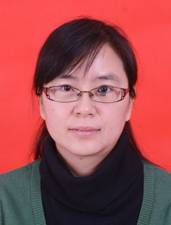马冰清
 发布时间： 2014-01-19   浏览次数： 6686姓名：马冰清 (Bingqing Ma)岗位职称：副教授研究方向：随机模型；微分几何电　　话：0373-3326148                                   传　　真：0373-3326174 电子邮箱：bqma@henannu.edu.cn 通信地址：河南师范大学数学与信息科学学院邮　　编：453007

 个人简历

1997.92001.6，河南师范大学数学系，本科

2003.92006.6，河南师范大学应用数学专业，硕士

2017.9—，河南师范大学数学物理专业，博士

2001.72003.8 商丘师范学院，助教

2006.72007.7 河南师范大学，助教

2007.72014.4 河南师范大学，讲师

2014.4—河南师范大学，副教授

 研究领域

 教学工作

 获奖情况

 科研项目

 论文著作

1. 概率论与数理统计，郑州：郑州大学出版社， 2007 ISBN 978-7-81106-506-0 (参编).

2. Searching for a counterfeit coin with b-balance, Discrete Applied Mathematics, 2006, 154: 2010-2023. (with W. Liu and H. Cui). SCI

3. Ricci-Hamiton流上特征值的单调性, 河南师范大学学报(自然科学版), 2007, 35: 30-32. (with G. Huang).

4. 紧致齐性黎曼流形上的特征值估计, 河南师范大学学报(自然科学版), 2008, 36: 9-11. (with G. Huang).

5. Gradient estimates for a nonlinear parabolic equation on Riemannian manifolds, Arch. Math. (Basel). 2010, 94: 265-275 (with G. Huang). SCI

6. Totally real sectional curvature for submanifolds in Sasakian space forms, Chinese Quart. J. Math., 2011, 26: 174-178 (with G. Huang).

7. Estimates on the first two poly-Laplacian eigenvalues on spherical domains, Acta Math. Sci. Ser. B Engl. Ed., 2012, 32B: 745-751. SCI

8. Gradient estimates for a nonlinear equation $\Delta_fu+du^{-\alpha}=0$ on complete noncompact manifolds, Commun. Math. 2011, 19: 73-84 (with J. Zhang).

9. Estimates for lower order eigenvalues of quadratic polynomials of the Laplacian, Arch. Math. (Basel). 2012, 98: 477-486 (with J. Zhang). SCI

10. 紧致黎曼流形上的Yamabe soliton, 河南师范大学学报(自然科学版), 2012, 40(6): 12-13. (with S. Yau).

11. Differential Harnack estimate for a nonlinear parabolic equation under List's flow, Comm. Math. Appl. 2013, 4(1): 75-83

12. 双曲空间上有关拉普拉斯二次多项式的特征值估计, 河南师范大学学报(自然科学版), 2013, 41(3): 26-28. (with X. Hou).

13. Eigenvalue relationships between Laplacians of constant mean curvature hypersurfaces in $S^{n+1}$, Commun. Math. 2013, 21: 31-38 (with G. Huang).

14. Lower bounds for the scalar curvature of noncompact gradient solitons of List’s flow, Arch. Math. (Basel). 2013, 100: 593-599 (with G. Huang). SCI

15. Estimates for lower order eigenvalues for operators in divergence form on Riemannian manifolds, J. Math.(PRC), 2013, 33(5): 761-766 (with J. Zhang).

16. The classification of gradient Ricci almost solitons, J. Math.(PRC), (with F. Zeng).

17. Some evolution equations under the List's flow and their applications, Comment. Math. Univ. Carolin. 2014, 55(1): 41-52

18. Eigenvalues of geometric operators under the List's flow, Comm. Math. Appl. 2013, 4(2): 127-135.

19. Gradient estimates of porous medium equations under the Ricci flow, J. Geom. 2014, 105(2): 313-325 (with J. Li).

20. Gradient estimates and Liouville type theorems for a nonlinear elliptic equation, Arch. Math. (Basel) 2015, 105(5): 491-499 (with G. Huang). SCI

21. Hamilton-Souplet-Zhang's gradient estimates for two types of nonlinear parabolic equations under the Ricci flow, J. Funct. Spaces 2016, Art. ID 2894207, 7 pp. (with G. Huang). SCI

22. Sharp bounds for the first nonzero Steklov eigenvalues for f-Laplacians, Turkish J. Math. 2016, 40(4): 770-783 (with G. Huang). SCI

23. Riemannian manifolds with harmonic curvature, Colloq. Math. 2016, 145(2): 251-257 (with G. Huang). SCI

24. Hamilton's gradient estimates of porous medium and fast diffusion equations, Geom. Dedicata 2017, 188: 1-16 (with G. Huang). SCI

25. Eigenvalue estimates for submanifolds with bounded f-mean curvature, Proc. Indian Acad. Sci. Math. Sci. 2017, 127(2): 375–381 (with G. Huang). SCI

26. Rigidity of complete noncompact Riemannian manifolds with harmonic curvature, J. Geom. Phys. 2018, 124: 233-240 (with G. Huang). SCI

27. Gradient estimates and Liouville-type theorems for a weighted nonlinear elliptic equation, J. Inequal. Appl. 2018, Paper No. 112, 10 pp. (with Y. Dong). SCI

28. Hamilton-Souplet-Zhang's gradient estimates and Liouville theorems for a nonlinear parabolic equation, C. R. Math. Acad. Sci. Paris 2018, 356(5): 550-557(with F. Zeng). SCI

29. Rigidity of Einstein metrics as critical points of quadratic curvature functionals on closed manifolds, Nonlinear Anal. 2018, 175: 237-248 (with G. Huang, X. Li and Y. Chen). SCI

30. Gradient estimates for a nonlinear elliptic equation on complete Riemannian manifolds, Proc. Amer. Math. Soc. https://doi.org/10.1090/proc/14106 (with G. Huang and Y. Luo). SCI